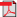# Search Results

1. E. Hyytiä and J. Virtamo, Dynamic Routing and Wavelength Assignment Using First Policy Iteration, in The Fifth Symposium on Computers and Communications (ISCC'00), pp. 146-151, 2000, Antibes - Juan Les Pins, France(bib)
Abstract: With standard assumptions the routing and wavelength assignment problem (RWA) can be viewed as a Markov Decision Process (MDP). The problem, however, defies an exact solution because of the huge size of the state space. Only heuristic algorithms have been presented up till now. In this paper we propose an approach where, starting from a given heuristic algorithm, one obtains a better algorithm by the first policy iteration. In order to estimate the relative costs of states, we make a simulation on the fly studying, at each decision epoch, the consequences of all the alternatives actions. Being computationally intensive, this method can be used in real time only for systems with slow dynamics. Off-line it can be used to assess how close the heuristic algorithms come to the optimal policy. Numerical examples are given about the policy improvement.